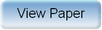Ninth International Geostatistics Congress, Oslo, Norway
June 11 – 15, 2012Session: Posters Abstract No.: P-045 Title: A New Stochastic Modeling Method for the 3-D Forecasting of IHS in Point Bar of Sinuous Channel Author(s): Yanshu Yin, Yangtze University (CN)Changmin Zhang, Yangtze University (CN)Shaohua Li, Yangtze University (CN) Abstract: The sinuous fluvial reservoir has very complex architectures due to the the well-known inclined heterolithic stratification(IHS) in point bar, which is proved to decide the distribution of the remaining oil by productive data in oilfield, and now analyzed and modeled by geologist. But the modeling of IHS is very difficult. The published Alluvsim can gives rather beautiful result, but can not be conditional in matured oilfield. Multiple point geostatistics improves the shape reproduction, but can not simulate the areal sinuous shape of IHS even by a large train image provided by Alluvsim. This paper proposed a new stochastic modeling method for IHS forecasting. The idea is based on the relationship of the channel curvature and the probability of the occurrence of point bar, and the relationship of the channel curvature, the geometric shape of point bar and the shape of IHS. As informed by outcrop ,modern sinuous fluvial sediment and water-basin experiment, The probability of the creation of point bar is primary decided by the curvature of the sinuous channel. A probability function of the occurrence of point bar is deduced. Then, the dip angle and orientation of the IHS is decided by the geometric shape and place of the point bar. The modeling strategy is hierarchical. First, the sinuous channel is constructed using Fluvsim and the curvature ratios of each channel are calculated for the determination of the probability of occurrence and placement of the point bar; then the point bar is placed by the occurrence probability. The geometric shape of point bar is characterized by the discarded channel in the sinuous channel ;finally the IHS in point bar is forecasted by the parameters such as the number, the areal shape, the dip and extended distance ,etc, which are deduced by the channel curvature ratio, the geometric shape of point bar. The inclined azimuth of IHS is pointed to the discarded channel rigorously. A real reservoir model in the eastern china are built using this method and the model has validated by newly drilled wells. The model error is less than 20 percent. The research both richened the theory of stochastic modeling ,and also provides a new method and technology for the enhancement of oil recovery.Produced by X-CD Technologies Inc.